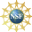# 8. Properties of Curves

## 2. Arc Length Parameter

Often, it is convenient to parametrize a curve with respect to its arc length. This is much more geometrical since it removes the arbitrary nature of the parameter. Physically, if the parameter is the time, then the particle may be speeding up and slowing down as it traverses the curve. If we switch to the arc length parameter, then we are traveling at unit speed, one unit of distance per unit of time.

Having found the formula for the arc length of a curve, it is easy to define the arclength parameter for the curve. We start with the formula we derived on the previous page but replace the upper limit by a variable point:

The arc length function starting from the point $$A=\vec r(a)$$ is the integral $s(t)=\int_A^{\vec{r}(t)} \,ds=\int_a^t |\vec{v}|\,dt$

The strategy is to solve this equation, $$s=s(t)$$, for $$t$$ as a function of $$s$$ and substitue $$t=t(s)$$ back into the original equation. This changes the parameter of the curve from the "time" $$t$$ to the "arc length" $$s$$. Unfortunately, this is usually quite difficult to do. Here is an example where it can be done:

Consider the helix $$\vec{r}(t)=(4\cos t,4\sin t,3t)$$. Find the velocity with respect to $$t$$, called $$\vec{v}_t$$, and its length, $$|\vec{v}_t|$$. Then reparametrize the curve with respect to arc length, $$s$$, starting from $$t=0$$. Finally, find the velocity with respect to $$s$$, called $$\vec{v}_s$$, and its length $$|\vec{v}_s|$$. (Since we will have $$2$$ parameters, we write $$\vec{v}_t$$ for the velocity with respect to $$t$$ and $$\vec{v}_s$$ for the velocity with respect to $$s$$.)

The velocity with respect to $$t$$ and its length are \begin{aligned} \vec{v}_t &=\dfrac{d\vec{r}(t)}{dt}=\langle -4\sin t,4\cos t,3\rangle \\ |\vec{v}_t| &=\sqrt{(-4\sin t)^2+(4\cos t)^2+3^2} \\ &=\sqrt{16(\sin^2t+\cos^2t)+9}=5 \end{aligned} The arclength function is $$\displaystyle s(t)=\int_0^t |\vec{v}|\,dt=\int_0^t 5\,dt=5t$$. So: $s=5t \qquad \text{and} \qquad t=\dfrac{s}{5}$ Substituting this back into the parametrized curve, we get (by ): $\vec{r}(s) =\left(4\cos\left(\dfrac{s}{5}\right),4\sin\left(\dfrac{s}{5}\right), \dfrac{3s}{5}\right)$ Finally, the velocity with respect to $$s$$ and its length are \begin{aligned} \vec{v}_s &=\dfrac{d\vec{r}(s)}{ds} =\left(-\,\dfrac{4}{5}\sin\left(\dfrac{s}{5}\right), \dfrac{4}{5}\cos\left(\dfrac{s}{5}\right), \dfrac{3}{5}\right) \\ |\vec{v}_s| &=\sqrt{\left(-\,\dfrac{4}{5}\sin\left(\dfrac{s}{5}\right)\right)^2 +\left( \dfrac{4}{5}\cos\left(\dfrac{s}{5}\right)\right)^2 +\dfrac{3}{5}^2} =\sqrt{\dfrac{16}{25}+\dfrac{9}{25}}=1 \end{aligned}

#### Abuse of Notation

Mathematicians would not like using $$\vec{r}(t)$$ for the curve as a function of $$t$$ and $$\vec{r}(s)$$ for the curve as a function of $$s$$. They say $$\vec r$$ should be the function and not change its formula when we plug in a different argument. However, physicists and engineers prefer a variable to refer to a physical or geometrical quantity, such as a parametric curve, or the time, or the arc length. So when we use $$\vec{r}(t)$$ and $$\vec{r}(s)$$ to refer to different functions, mathematicians refer to it as "abuse of notation". We need to know what is meant from the context.

On the next page, you will learn that the length of the velocity is the speed the particle traverses the curve. So it is no surprise that, using the arc length parameter as the time, the speed is $$1$$, one unit of distance per unit of time.

The arc length parameter, $$s$$ is very useful when discussing the geometry of the curve. On the other hand, $$t$$ is more useful for analyzing physical situations.

The integral in the definition of $$s(t)$$ is more often than not impossible to do, and even if you can do the integral it is unlikely that you can solve for $$t(s)$$. Problems in this textbook and on tests are selected from the small collection of curves that will make the integral doable and the equation solvable. Here is an exercise where the reparametrization can be done followed by an example where it cannot be done.

Consider the circle $$\vec{r}(\theta)=(R\cos\theta,R\sin\theta,3)$$. Find the velocity with respect to $$\theta$$, called $$\vec{v}_{\theta}$$, and its length, $$|\vec{v}_{\theta}|$$. Then reparametrize the curve with respect to arc length, $$s$$, starting from $$\theta=0$$. Finally, find the velocity with respect to $$s$$, called $$\vec{v}_s$$, and its length $$|\vec{v}_s|$$.

\begin{aligned} \vec{v}_{\theta} &=\langle -R\sin\theta,R\cos\theta,0\rangle \\ |\vec{v}_{\theta}| &=R \\ s&=R\theta \qquad \theta=\frac{s}{R} \\ \vec{r}(s) &=\left(R\cos\left(\dfrac{s}{R}\right),R\sin\left(\dfrac{s}{R}\right),3\right) \\ \vec{v}_s &=\left\langle -\sin\left(\dfrac{s}{R}\right), \cos\left(\dfrac{s}{R}\right),0\right\rangle \\ |\vec{v}_s| &=1 \end{aligned}

The velocity with respect to $$\theta$$ and its length are \begin{aligned} \vec{v}_{\theta} &=\langle -R\sin\theta,R\cos\theta,0\rangle \\ |\vec{v}_{\theta}| &=\sqrt{(-R\sin\theta)^2+(R\cos\theta)^2} \\ &=\sqrt{R^2(\sin^2\theta+\cos^2\theta)}=R \end{aligned} The arclength is $s(\theta)=\int_0^{\theta} |\vec{v}|\,d\theta =\int_0^{\theta} R\,d\theta=R\,\theta$ So: $s=R\theta \qquad \text{and} \qquad \theta=\dfrac{s}{R}$ Substituting this back into the parametrized curve, we get $\vec{r}(s) =\left(R\cos\left(\dfrac{s}{R}\right),R\sin\left(\dfrac{s}{R}\right),3\right)$ Finally, the velocity with respect to $$s$$ and its length are \begin{aligned} \vec{v}_s &=\left\langle -\sin\left(\dfrac{s}{R}\right), \cos\left(\dfrac{s}{R}\right),0\right\rangle \\ |\vec{v}_s| &=\sqrt{\sin^2\left(\dfrac{s}{R}\right) +\cos^2\left(\dfrac{s}{R}\right)}=1 \end{aligned}

Reparameterize the twisted cubic $$\vec{r}(t)=\left(t,t^2, \dfrac{2}{3}t^{3}\right)$$with respect to arclength. Then find its velocity, $$\vec v_s$$, and the length of the velocity, $$|\vec v_s|$$.

We want to find $$\displaystyle s(t)=\int_0^t |\vec{v}|\,dt$$. Previously, we found the velocity is $$\vec{v}=\left\langle 1,2t,2t^2\right\rangle$$ and its length is $$|\vec{v}|=1+2t^2$$
Plugging this in gives: $s(t)=\int_0^t (1+2t^2)\,dt=t+\dfrac{2}{3}t^{3}$ This expression cannot easily be solved for $$t.$$ Thus, the curve cannot easily be reparameterized by $$s$$. However, we can still think about the curve parametrized by $$s$$ as $$\vec{r}(s)=\left(t(s),t(s)^2,\dfrac{2}{3}t(s)^3\right)$$ even if we cannot write it down. The new velocity is found using the Chain Rule: \begin{aligned} \vec v_s&=\left(\dfrac{dt}{ds},2t(s)\dfrac{dt}{ds},2t(s)^2\dfrac{dt}{ds}\right) \\ &=\dfrac{1}{\;\frac{\small ds}{\small dt}\;}\left(1,2t(s),2t(s)^2\right) \\ &=\dfrac{1}{|\vec v_t|}\left(1,2t(s),2t(s)^2\right) \\ &=\dfrac{1}{1+2t(s)^2}\left(1,2t(s),2t(s)^2\right) \\ \end{aligned} where we have recognized $$\dfrac{ds}{dt}$$ as the length of the original velocity, $$\vec v_t$$. Then the length is: $|\vec v_s|=\dfrac{1}{1+2t(s)^2}\sqrt{1+4t(s)^2+4t(s)^4} =\dfrac{1+2t(s)^2}{1+2t(s)^2}=1$

Supported in part by NSF Grant #1123255